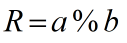Request a Tool

Modulo Calculator

Modulo Calculator is use to calculate remainder from dividion of dividend and divisor.

Remainder
0

Formula• R = Remainder
• a = Values of Dividend
• b = Values of Divisor

Defination / Uses

When one integer is split by another, the modulo operation returns the remainder or signed remainder. The remainder of the Euclidean division of a by n, where an is the dividend and n is the divisor, is a modulo n, given two positive numbers a and n.

Addition, subtraction, multiplication, and division are all terms that we are all familiar with. These are the basic mathematical operations that we were taught in elementary school, and their operators, +, -, *, and /, are all well-known. So, how does the modulus operator work? The modulus operator, often known as percent or mod in most programming languages, performs modulo operations. When computing the mod of an integer, our basic modulo calculator takes these operators into account. Keep in mind that mod isn't looking for the division's outcome; rather, it's looking for what's left. In computer operations, the modulo operation is extremely useful.